# Forms

##### Basic Definitions

A p-form is a type (0,p) tensorthat is totally antisymmetric: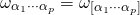The square brackets denote antisymmetrization; for example,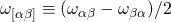. The exterior product (wedge product) of a p-form and a q-form is a p+q form:If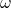andare both 1-forms,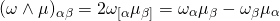The exterior derivative of a p-formis a p+1 form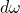defined by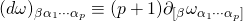Any derivative operator can be used in place of the coordinate derivative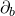. Note that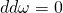. Ifis a p-form andis a q-form,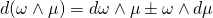where the plus sign applies if p is even, the minus sign applies if p is odd.

##### INTEGRATION

If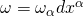and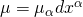are both 1-forms, thenor, in index-free notation,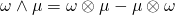Let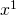,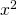, etc. denote right handed coordinates on an n-dimensional manifold. Thus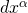are 1-forms and we have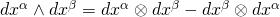. Extending to a multiple product of 1-forms,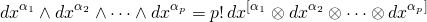Expand the general p-formin the coordinate basis:For an n-form in particular,The integral of the n-form is defined byA volume element is a nonvanishing n-form. It can be used to define the integral of a function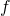by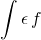##### STOKES’ THEOREM

Let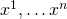be a right handed coordinate system on an n-dimensional manifold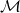with boundary. Let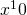define the exterior. Then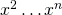is a right handed coordinate system on the n-1 dimensional boundary. Stokes theorem says that for an n-1 form,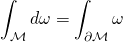##### LEVI-CIVITA TENSOR IN 4D

Letbe a 4-dimensional spacetime manifold with metric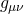and signature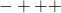. The natural volume element is the Levi-Civita tensor defined by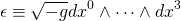where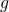is the determinant of the metric and the coordinates are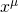with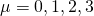. This volume element has components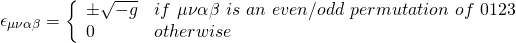and it satisfies

(1)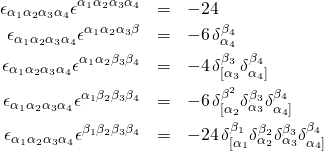For an element of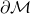, the 3-dimensional boundary of, the  natural volume element  is defined by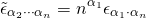where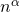is the outward pointing unit normal. If follows that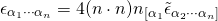where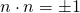depending on whetheris spacelike or timelike.

##### LEVI-CIVITA TENSOR IN 3D

Let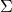be a 3-dimensional spacetime manifold with metric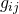and signature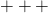. The natural volume element is the Levi-Civita tensor defined by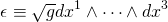whereis the determinant of the metric and the coordinates are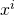with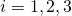. This volume element has components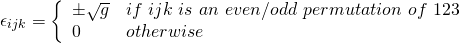and it satisfies

(2)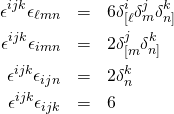##### STOKES’ THEOREM AGAIN

In terms of natural volume elements, Stokes’ theorem reduces towhere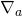is the covariant derivative compatible with the metric andis a contravariant vector. The integral on the right-hand side must be summed over all elements of the boundary. This relation is written in coordinates as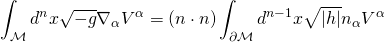where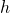is the determinant of the induced metric on.

##### STOKES’ THEOREM IN TWO DIMENSIONS

Let the dimension of the manifold be n=2. Assume the metric has signature ++. Let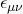denote the Levi–Civita tensor onand write the vector fieldin terms of a covector field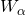as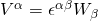. In this case Stokes’ theorem reduces toThis is what Jackson calls “Stokes’ theorem”. In this context, the two dimensional manifold is a surface embedded in three dimensional space and the two dimensional Levi-Civita tensor is equal to the three dimensional Levi-Civita tensor with one index contracted with the unit normal to the surface. The result above relates the curl of a vector field, integrated over a surface, to the circulation on the boundary.